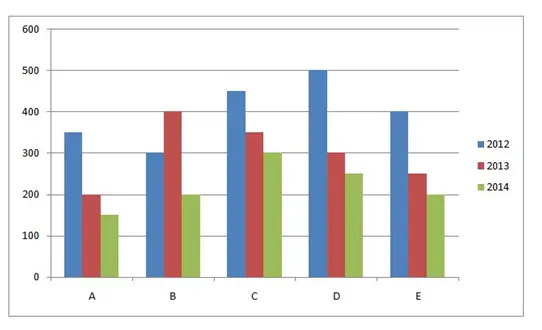# SBI Clerk Prelims 2021 Quantitative Aptitude Questions (Day-50)

Dear Aspirants, Our IBPS Guide team is providing new series of Quantitative Aptitude Questions for SBI Clerk Prelims 2020 so the aspirants can practice it on a daily basis. These questions are framed by our skilled experts after understanding your needs thoroughly. Aspirants can practice these new series questions daily to familiarize with the exact exam pattern and make your preparation effective.

Start Quiz

Approximations

Directions (01-05): What approximate value should come in place of question mark in the following questions?

1) 79.940 % of 39.892 + √224.918 * 4.902 + 89.923 % of 19.928=?

A.125

B.120

C.115

D.110

E.130

2) 39.189 ÷ 12.943 + 49.891 * 1.904 + 1.034 * 6.921= ? * 10.941

A.7

B.10

C.14

D.18

E.3

3) 74.912 % of 7.912 + ? * 16.902 = √5040 * 1.903

A.8

B.12

C.16

D.20

E.4

4) 5.9012 * 9.823 + 87.897 ÷ 7.902 + 2.908 * 12.908=?

A.100

B.110

C.120

D.90

E.130

5) 199.903 –14.903 * 6.123 + 34.908 * 8.319 =?

A.330

B.360

C.390

D.420

E.450

Bar graph

Directions (06-10): Study the following information carefully and answer the given questions:

The given bar graph shows the number of mobile manufactured in three different years in five different companies.6) Total number of mobile manufactured in 2012 in all the companies together in approximately what percent more than the total number of mobile manufactured from all the companies together in 2013?

A.33.33%

B.31.11%

C.35.56%

D.37.77%

E.30.03%

7) In 2014 how many companies manufactured less than 25% of the total number of mobile manufactured in the given period?

A.0

B.3

C.4

D.2

E.1

8) If the cost price of one mobile in 2012 and 2014 is Rs.6000 and Rs.8000 respectively, what is the difference between the total revenue collected in company B in 2014 and company C in 2012?

A.6 lakh

B.8 lakh

C.9 lakh

D.11 lakh

E.12 lakh

9) What is the difference between the total number of mobile manufactured from E in all the years together and the total number of mobile manufactured from B in all the years together?

A.20

B.50

C.100

D.200

E.150

10) What is the average number of mobile manufactured in 2014 from all the companies together?

A.100

B.150

C.240

D.220

E.180

79.940 % of 39.892 + √224.918 * 4.902 + 89.923 % of 19.928=?

80% of 40 + √225 * 5 + 90% of 20 = ?

32 + 75 + 18=?

125=?

39.189 ÷ 12.943 + 49.891 * 1.904 + 1.034 * 6.921= ? * 10.941

39/13 + 50 * 2 + 1 * 7 = ? * 11

3 + 100 + 7=? * 11

?=10

74.912 % of 7.912 + ? * 16.902 = √5040 * 1.903

75% of 8 + ? * 17 = √5041 * 2

6 + ? * 17=142

?=8

5.9012 * 9.823 + 87.897 ÷ 7.902 + 2.908 * 12.908=?

60 + 11 + 39=?

110=?

199.903 – 14.903 * 6.123 + 34.908 * 8.319 =?

200 – 15 * 6 + 35 * 8 = ?

200 – 90 + 280=?

390=?

Total number mobile manufactured in 2012=300 + 350 + 400 + 450 + 500

=2000

Total number of mobile manufactured in 2013=200 + 250 + 300 + 350 + 400

=1500

Required percentage=[(2000 – 1500)/1500] * 100=33.33%

Total number of mobile manufactured in A=350 + 200 + 150=700

Required percentage=150/700 * 100=21.42%

Total number of mobile manufactured in B=300 + 400 + 200=900

Required percentage=200/900 * 100=22.22%

Total number of mobile manufactured in C=450 + 350 + 300=1100

Required percentage=300/1100 * 100=27.27%

Total number of mobile manufactured in D=500 + 300 + 250=1050

Required percentage=250/1050 * 100=23.809%

Total number of mobile manufactured in E=400 + 250 + 200=850

Required percentage=200/850 * 100=23.52%

Required difference=6000 * 450 – 8000 * 200=1100000

Difference= (300 + 400 + 200) – (400 + 250 + 200) =50